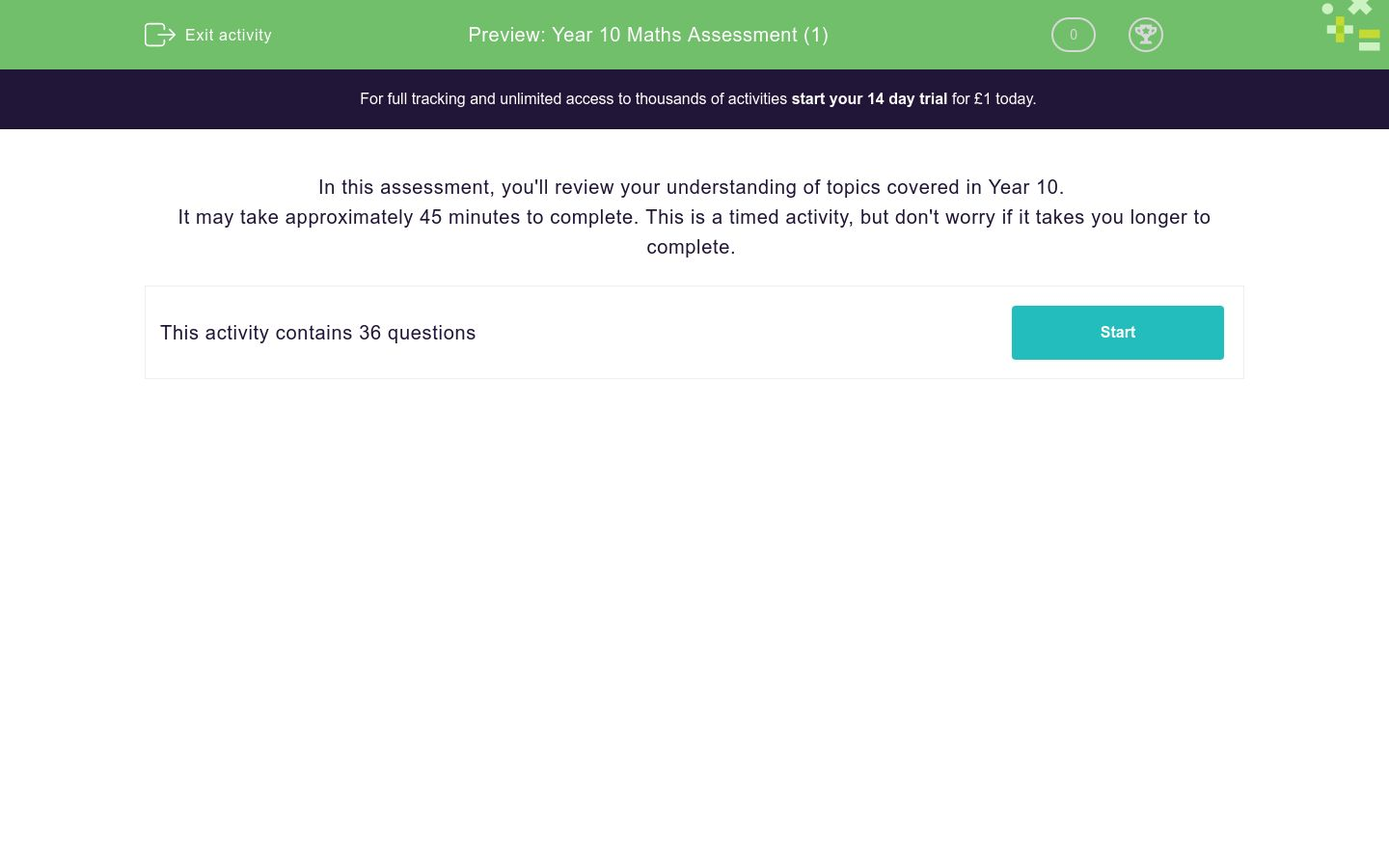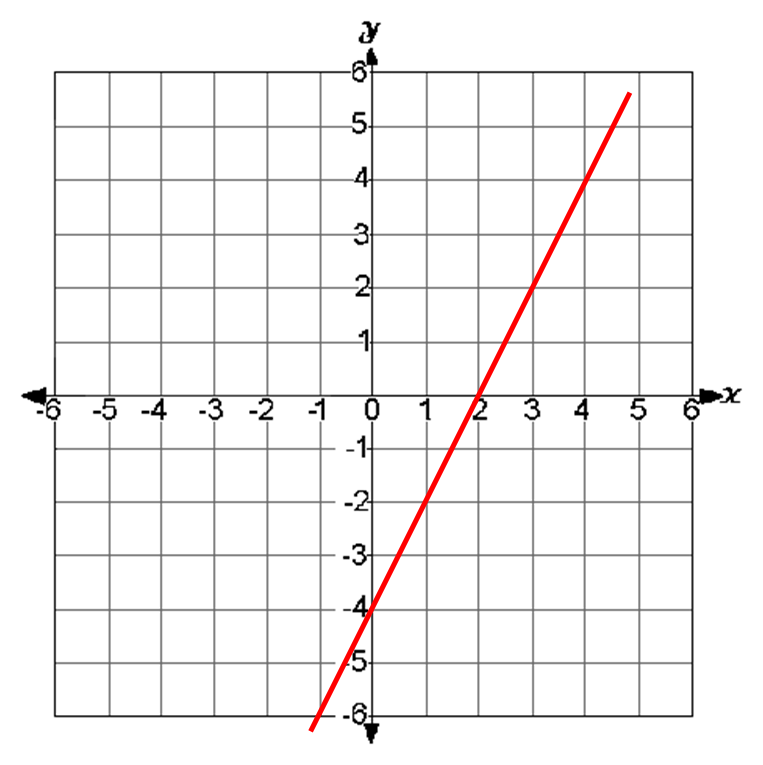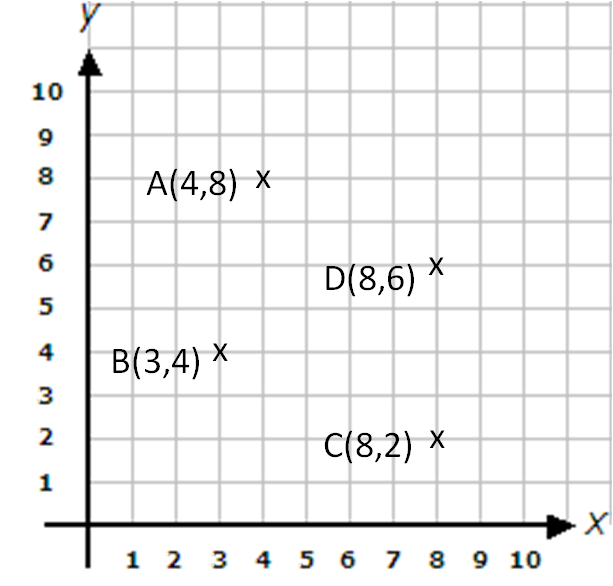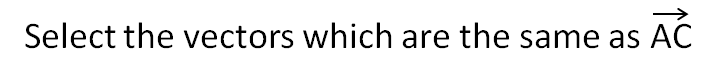# Year 10 Maths Assessment (1)

In this assessment, students will review their comprehension of topics covered in Year 10. May take approximately 45 minutes to complete.Key stage:  KS 4

Curriculum topic:  Assessment

Curriculum subtopic:  Starter Assessment

Difficulty level:### QUESTION 1 of 10

In this assessment, you'll review your understanding of topics covered in Year 10.

It may take approximately 45 minutes to complete. This is a timed activity, but don't worry if it takes you longer to complete.

Round the following number to 1 significant figure.

275

Select the answer which is equal to:

16½

8

16½

4

Select the answer which is equal to:

5-1

-5

0.2

Multiply together

4 x 106  x   6 x 104

(The answer must be in standard form.)

24 x 1010

2.4 x 1011

2.4 x 1025

Divide

4 x 106  ÷   2 x 104

(The answer must be in standard form.)

2 x 101.5

2 x 102

8 x 1010

Jack invests £200 at 2% interest p.a.

How much interest is earned after one year?

A bar of chocolate is bought for £1.20 and sold for £1.44.

Calculate the percentage profit.

(Just write the number as a whole number or to 3 sig. figs. if not whole.)You may use a calculator for this question.

Use a percentage multiplier to calculate the original price.

A child's car seat costs £68.25 after 5% VAT has been added. What was its price before VAT?

£65

£68.25

£61.75

£65.50You may use a calculator for this question.

A piece of chromium has a mass of 50g and a volume of 6.95 cm3.

Find the density of chromium in g/cm3.

(Just write the number to 3 sig. figs.)

A car travels the 36 miles from Liverpool to Manchester in ¾ hour.

Find the average speed in mph.

(Just write the number.)

Simplify

3(4a - 2) + (2a + 5)

Multiply out the brackets:

(3a + 2) (12a + 5)

36a2+39a +10

36a2+3a +10

15a2+39a +2

a2+17a +10

Factorise the following quadratic expression in pairs and then fully.

3a2 + 18a + 2a + 12

(3a + 2) (a + 6)

(a + 1) (5a + 6)

(2a + 3) (3a + 2)

(3a + 2) (3a + 4)

x2 + 6x - 16

(x - 7) (x - 6)

(x + 6) (x - 7)

(x - 8) (x - 2)

(x - 2) (x + 8)You may use a calculator for this question.

x2 + 7x + 9 = 0

x = -5.30

x = 1.70

x = -1.70

x = 5.30

Solve simultaneously:

3x + 7y = 0

x - y = 10

x = 3, y = 7

x = 7, y = -3

x = 21, y = 1

Find the gradient of the straight line which passes through:

(1, 5) and (5, 9)

(If it is not a whole number, write it as a fraction in the form a/b.)

State the gradient of the line with equation:

 y - 3 = x 2

Which of the given equations represents the red line shown below?y = 2x - 4

x = 2y + 4

y = 2x + 2

y = -4x + 2

Solve the following equation for a:

 3a + 2a = 12 2 4

(Just write the value of a.)

Simplify the following and select the correct answer:

 a + 2 - a + 5 10 15

 1 3 2. a - 4 3. 2a + 7 15 30 25

1.

2.

3.

Find three consecutive numbers whose sum is 33.

Using x for the smallest number, select the equation which fits the given information.

x + x + 1 + x + 2 = 33

x + x + 2 + x + 4 = 33

x + x + x = 33

Find the value of x from the information given in this diagram.(not drawn to scale)

Find the number of sides in a polygon whose interior angle sum is 540º.

Select all the Pythagorean Triads from the list below.

(Remember to look out for multiples too!)

3, 4, 5

4, 5, 6

5, 6, 9

6, 8, 10

In the following diagram, work out the bearing of B from A .x = 40°
Bearing of B from A =
 ?

Just write the number in the text box below.You may use a calculator for this question.

Find the circumference of a circle with radius 5 cm.

Just write the number in the box below. Don't forget to round to 3 sig. figs.You may use a calculator for this question.

Calculate the arc length of the given shape to 3 sig. figs.

 Remember, arc length = 2 × r ××for radius r, angle at centre =360R = 24 cm

Θ = 11.5 °

Arc length = ____ cm

Triangle ABC has a right angle at B.

AB = 10 cm

∠ACB = 22.5º

After sketching the triangle, use trigonometry to calculate the length of the side BC.

(Give your answer to 3 sig. figs. and just write the number.)

The diagram shows the side of a shed against a vertical wall.

Find the width of the side of the shed in metres to 3 sig. figs.

(Just write the number.)In the diagram,

A is at (4,8); B is at (3,4); C is at (8,2); D is at (8,6)

State the vector which maps B to A.1 ( 1 ) 2. ( -5 ) 3. ( 4 ) 4 2 -6

1.

2.

3.

 ( 4 ) + ( -2 ) 5 5

 1 ( 6 ) 2. ( 4 ) 3. ( 2 ) 10 10 10

1.

2.

3.q + r

q - r

p - s

A bag contains 4 red counters, 5 blue counters and 6 yellow counters.

One counter is selected at random.

What is the probability that it is yellow or blue?

(Write your answer as a fraction in its lowest terms in the form a/b.)

A box of chocolates contains 5 dark chocolates, 2 white chocolates and 3 toffees.

One item is selected at random and then replaced. A second item is then selected.

What is the probability that a dark chocolate is chosen followed by a toffee?

(Write your answer as a fraction in its lowest terms in the form a/b.)

Six counters numbered 2, 4, 5, 7, 8, 9 are placed in a bag.

If one counter is drawn at random, what is the probability that it will be:

(a) a factor of 35

(b) even?

## Column B

Factor of 35
1/3
Even
1/2
• Question 1

Round the following number to 1 significant figure.

275

300
EDDIE SAYS
275 rounds up to 300 because it is closer to 300 than it is to 200.
• Question 2

Select the answer which is equal to:

16½

4
EDDIE SAYS
A power to the ½ is the same as a square root.
• Question 3

Select the answer which is equal to:

5-1

0.2
EDDIE SAYS
5-1 = 1/5 = 0.2
• Question 4

Multiply together

4 x 106  x   6 x 104

(The answer must be in standard form.)

2.4 x 1011
EDDIE SAYS
24 × 1010 is not standard form.
• Question 5

Divide

4 x 106  ÷   2 x 104

(The answer must be in standard form.)

2 x 102
EDDIE SAYS
4 ÷ 2 = 2
106 ÷ 104 = 102
• Question 6

Jack invests £200 at 2% interest p.a.

How much interest is earned after one year?

4.00
4
EDDIE SAYS
2 ÷ 100 × 200
• Question 7

A bar of chocolate is bought for £1.20 and sold for £1.44.

Calculate the percentage profit.

(Just write the number as a whole number or to 3 sig. figs. if not whole.)

20
EDDIE SAYS
24 ÷ 120 × 100
• Question 8You may use a calculator for this question.

Use a percentage multiplier to calculate the original price.

A child's car seat costs £68.25 after 5% VAT has been added. What was its price before VAT?

£65
EDDIE SAYS
£68.25 ÷ 1.05
• Question 9You may use a calculator for this question.

A piece of chromium has a mass of 50g and a volume of 6.95 cm3.

Find the density of chromium in g/cm3.

(Just write the number to 3 sig. figs.)

7.19
EDDIE SAYS
50 ÷ 6.95
• Question 10

A car travels the 36 miles from Liverpool to Manchester in ¾ hour.

Find the average speed in mph.

(Just write the number.)

48
EDDIE SAYS
36 ÷ ¾ = 36 × 4/3
• Question 11

Simplify

3(4a - 2) + (2a + 5)

14a-1
EDDIE SAYS
12a - 6 + 2a + 5
• Question 12

Multiply out the brackets:

(3a + 2) (12a + 5)

36a2+39a +10
EDDIE SAYS
3a(12a + 5) + 2(12a + 5) 36a2 + 15a + 24a + 10
• Question 13

Factorise the following quadratic expression in pairs and then fully.

3a2 + 18a + 2a + 12

(3a + 2) (a + 6)
EDDIE SAYS
3a(a + 6) + 2(a + 6)
• Question 14

x2 + 6x - 16

(x - 2) (x + 8)
EDDIE SAYS
Look for factor pairs of -16 that add to give +6,
i.e. 8 and -2.
Use these to split the x term to get:
x2 + 8x - 2x -16
Factorise in pairs to get:
x(x + 8) - 2(x + 8)
• Question 15You may use a calculator for this question.

x2 + 7x + 9 = 0

x = -5.30
x = -1.70
EDDIE SAYS
In the quadratic formula, use a=1 b=7 c=9.
• Question 16

Solve simultaneously:

3x + 7y = 0

x - y = 10

x = 7, y = -3
EDDIE SAYS
Rearrange second equation to read x = 10 + y and substitute into the first equation to get:
3(10 + y) + 7y = 0
30 + 3y + 7y = 0
10y = -30
y = -3
Use second equation to get:
x = 10 + y = 10 - 3 = 7
• Question 17

Find the gradient of the straight line which passes through:

(1, 5) and (5, 9)

(If it is not a whole number, write it as a fraction in the form a/b.)

1
EDDIE SAYS
(9 - 5) ÷ (5 - 1)
• Question 18

State the gradient of the line with equation:

 y - 3 = x 2

2
EDDIE SAYS
Multiply both sides by 2 to get:
y - 3 = 2x
y = 2x + 3
• Question 19

Which of the given equations represents the red line shown below?y = 2x - 4
EDDIE SAYS
Intercept on y-axis is -4.
• Question 20

Solve the following equation for a:

 3a + 2a = 12 2 4

(Just write the value of a.)

6
EDDIE SAYS

l.c.m. is 4.

 4 x 3a + 4 x 2a = 4 x 12 2 4

Then simplify

2 x 3a + 2a = 48

• Question 21

Simplify the following and select the correct answer:

 a + 2 - a + 5 10 15

 1 3 2. a - 4 3. 2a + 7 15 30 25

2.
EDDIE SAYS
Change to 30ths.
On top you will have 3(a + 2) - 2 (a + 5)
i.e. 3a + 6 - 2a - 10
or a - 4.
• Question 22

Find three consecutive numbers whose sum is 33.

Using x for the smallest number, select the equation which fits the given information.

x + x + 1 + x + 2 = 33
EDDIE SAYS
The three consecutive numbers will be x, x + 1 and x + 2. Add them to get 33.
• Question 23

Find the value of x from the information given in this diagram.(not drawn to scale)

10
EDDIE SAYS
Scale factor = 9 ÷ 4.5 = 2
x = 5 × 2 = 10
• Question 24

Find the number of sides in a polygon whose interior angle sum is 540º.

5
EDDIE SAYS
540 ÷ 180 + 2
• Question 25

Select all the Pythagorean Triads from the list below.

(Remember to look out for multiples too!)

3, 4, 5
6, 8, 10
EDDIE SAYS
6, 8, 10 is double 3, 4, 5.
• Question 26

In the following diagram, work out the bearing of B from A .x = 40°
Bearing of B from A =
 ?

Just write the number in the text box below.

140
EDDIE SAYS
180 - 40 = 140
• Question 27You may use a calculator for this question.

Find the circumference of a circle with radius 5 cm.

Just write the number in the box below. Don't forget to round to 3 sig. figs.

31.4
EDDIE SAYS
2 × π × 5
• Question 28You may use a calculator for this question.

Calculate the arc length of the given shape to 3 sig. figs.

 Remember, arc length = 2 × r ××for radius r, angle at centre =360R = 24 cm

Θ = 11.5 °

Arc length = ____ cm

4.82
EDDIE SAYS
 2 × 24 ×× 11.5 360
• Question 29

Triangle ABC has a right angle at B.

AB = 10 cm

∠ACB = 22.5º

After sketching the triangle, use trigonometry to calculate the length of the side BC.

(Give your answer to 3 sig. figs. and just write the number.)

24.1
EDDIE SAYS
10/tan22.5°
• Question 30

The diagram shows the side of a shed against a vertical wall.

Find the width of the side of the shed in metres to 3 sig. figs.

(Just write the number.)2.75
EDDIE SAYS
1/tan20°
• Question 31

In the diagram,

A is at (4,8); B is at (3,4); C is at (8,2); D is at (8,6)

State the vector which maps B to A.1 ( 1 ) 2. ( -5 ) 3. ( 4 ) 4 2 -6

1.
EDDIE SAYS
Right 1 Up 4
• Question 32

 ( 4 ) + ( -2 ) 5 5

 1 ( 6 ) 2. ( 4 ) 3. ( 2 ) 10 10 10

3.
EDDIE SAYS
4 - 2 on the top.
5 + 5 on the bottom.
• Question 33q + r
p - s
EDDIE SAYS
q followed by r
p followed by a backwards s
• Question 34

A bag contains 4 red counters, 5 blue counters and 6 yellow counters.

One counter is selected at random.

What is the probability that it is yellow or blue?

(Write your answer as a fraction in its lowest terms in the form a/b.)

11/15
EDDIE SAYS
11 are yellow or blue out of 15 in total.
• Question 35

A box of chocolates contains 5 dark chocolates, 2 white chocolates and 3 toffees.

One item is selected at random and then replaced. A second item is then selected.

What is the probability that a dark chocolate is chosen followed by a toffee?

(Write your answer as a fraction in its lowest terms in the form a/b.)

3/20
EDDIE SAYS
5/10 × 3/10 = 15/100 which reduces.
• Question 36

Six counters numbered 2, 4, 5, 7, 8, 9 are placed in a bag.

If one counter is drawn at random, what is the probability that it will be:

(a) a factor of 35

(b) even?

## Column B

Factor of 35
1/3
Even
1/2
EDDIE SAYS
The counters numbered with factors of 35 are 7 and 5, which is one third of the total number of counters in the bag.
Half of the counters in the bag are numbered with even numbers, so the probability of drawing one of them is 1/2.
---- OR ----

Sign up for a £1 trial so you can track and measure your child's progress on this activity.

### What is EdPlace?

We're your National Curriculum aligned online education content provider helping each child succeed in English, maths and science from year 1 to GCSE. With an EdPlace account you’ll be able to track and measure progress, helping each child achieve their best. We build confidence and attainment by personalising each child’s learning at a level that suits them.